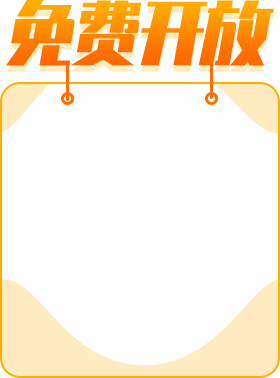# PHP培训之数组

//使用数组来完成案例

\$hens=3;

\$hens=5;

\$hens=1;

\$hens=3.4;

\$hens=2;

\$hens=50;

\$hens=30;

\$allWeight=0;

//遍历整个数组

//有时为了知道某个数组共有多少个元素,可以使用系统函数count

echo "共有".count(\$hens)."只鸡<br/>";

for(\$i=0;\$i<count(\$hens);\$i++){

echo "第".(\$i+1)."只鸡体重=".\$hens[\$i]."<br/>";

\$allWeight+=\$hens[\$i];

}

echo '<br/>总体重是='.\$allWeight.'平均体重='.\$allWeight/count(\$hens);

① 创建数组

\$arr=123;

\$arr=90;

\$arr=8;